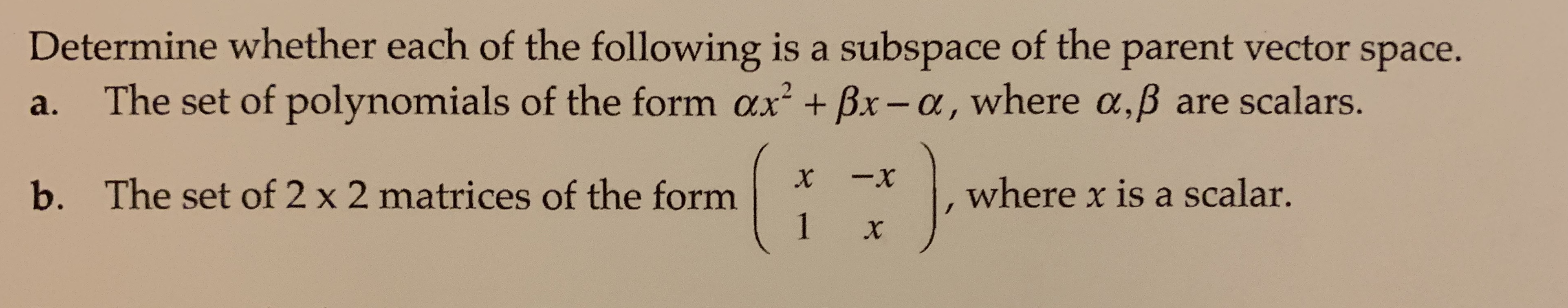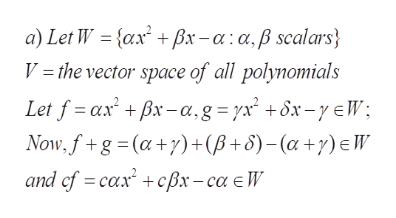Determine whether each of the following is a subspace of the parent vector space.The set of polynomials of the form ax + Bx-a, where a,B are scalars.2а.-xXThe set of 2 x 2 matrices of the form1where x is a scalar.b.X

Questionhelp_outlineImage TranscriptioncloseDetermine whether each of the following is a subspace of the parent vector space. The set of polynomials of the form ax + Bx-a, where a,B are scalars. 2 а. -x X The set of 2 x 2 matrices of the form 1 where x is a scalar. b. X fullscreen
Step 1

To determine whether the given subsets are subspaces

Step 2

(a) In this case W is a subspace of the parent vector space V, ...help_outlineImage Transcriptionclosea) Let W = {ax + Bx-a:a,ß scalars V = the vector space of all polynomials Let f axBx-a,g=rx +ôx-yeW; Now, fg (a)+(B+S)-(a+y) E W and cf caxcx-ca e V fullscreen

Want to see the full answer?

See Solution

Want to see this answer and more?

Our solutions are written by experts, many with advanced degrees, and available 24/7

See Solution
Tagged in

Math